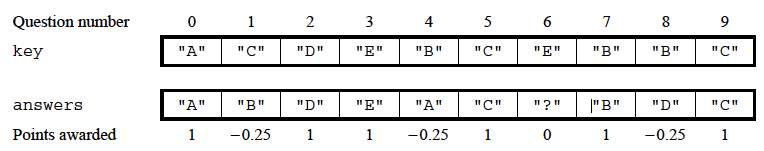# 16.5. StudentAnswerSheet - Part A¶

The following is a free response question from 2007. It was question 3 on the exam. You can see all the free response questions from past exams at https://apstudent.collegeboard.org/apcourse/ap-computer-science-a/exam-practice.

Question 3. Consider a system for processing student test scores. The following class will be used as part of this system and contains a student’s name and the student’s answers for a multiple-choice test. The answers are represented as strings of length one with an omitted answer being represented by a string containing a single question mark (`"?"`). These answers are stored in an `ArrayList` in which the position of the answer corresponds to the question number on the test (question numbers start at 0). A student’s score on the test is computed by comparing the student’s answers with the corresponding answers in the answer key for the test. One point is awarded for each correct answer and 1/4 of a point is deducted for each incorrect answer. Omitted answers (indicated by `"?"`) do not change the student’s score.

```public class StudentAnswerSheet
{

/** @param key the list of correct answers, represented as strings
*         of length one
*  Precondition: key.size() is equal to the number of answers in
*  @return this student's test score
public double getScore(List<String> key)
{
/* to be implemented in part (a) */
}

/** @return the name of the student
*/
public String getName()
{
/* implementation not shown */
}

// There may be other fields, constructors, and methods

}
```

The following table shows an example of an answer key, a student’s answers, and the corresponding point values that would be awarded for the student’s answers. In this example, there are six correct answers, three incorrect answers, and one omitted answer. The student’s score is ((6 * 1) - (3 * 0.25)) = 5.25.Part a. Write the `StudentAnswerSheet` method `getScore`. The parameter passed to method `getScore` is a `List` of strings representing the correct answer key for the test being scored. The method computes and returns a `double` that represents the score for the student’s test answers when compared with the answer key. One point is awarded for each correct answer and 1/4 of a point is deducted for each incorrect answer. Omitted answers (indicated by `"?"`) do not change the student’s score.
Complete method `getScore` below.
The code below has a main method for testing the `getScore` method.